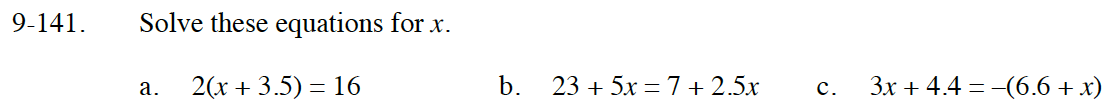Home > CC3 > Chapter 9 > Lesson 9.2.6 > Problem9-141

9-141.
1. Solve these equations for x. Homework Help ✎

1. 2(x + 3.5) = 16

2. 23 + 5x = 7 + 2.5x

3. 3x + 4.4 = −(6.6 + x)Distribute the 2.

x = 4.5

Move all variables to one side of the equation and all constants to the other side of the equation.

Remember to distribute the negative sign to the terms inside the parentheses.
3x + 4.4 = −6.6 − x

Add x to both sides and subtract 4.4 from both sides to isolate x.
4x = −11

x = −2.75